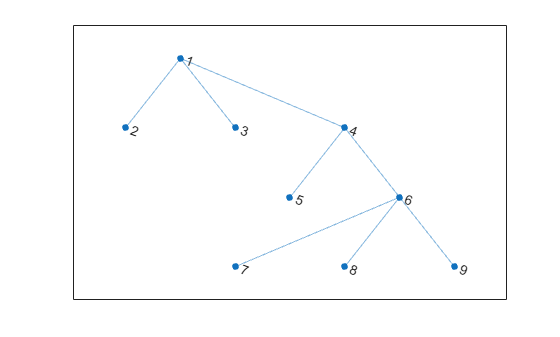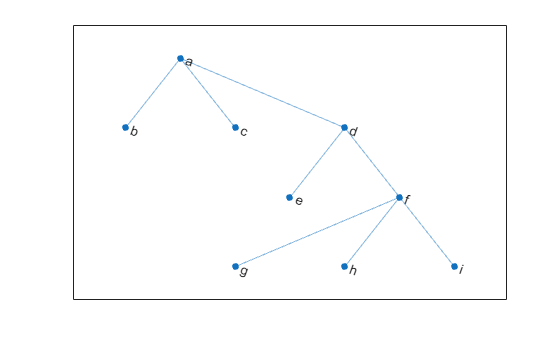degree

Degree of graph nodes

Description

example

D = degree(G) returns the degree of each node in graph G. The degree is the number of edges connected to each node.

example

D = degree(G,nodeIDs) returns the degree of the nodes specified by nodeIDs.

Examples

collapse all

Create and plot a graph, and then use degree to find the degree of each node in the graph.

s = [1 1 1 4 4 6 6 6];
t = [2 3 4 5 6 7 8 9];
G = graph(s,t);
plot(G)deg = degree(G)
deg = 9×1

3
1
1
3
1
4
1
1
1

deg(j) indicates the degree of node j.

Create and plot a graph, and then find the degree of the first, third, and fifth nodes.

s = {'a' 'a' 'a' 'd' 'd' 'f' 'f' 'f'};
t = {'b' 'c' 'd' 'e' 'f' 'g' 'h' 'i'};
G = graph(s,t);
plot(G)nodeIDs = {'a' 'c' 'e'}';
deg = degree(G,nodeIDs)
deg = 3×1

3
1
1

deg(j) indicates the degree of node nodeIDs(j).

Input Arguments

collapse all

Input graph, specified as a graph object. Use graph to create an undirected graph object.

Example: G = graph(1,2)

Node identifiers, specified as one or more node indices or node names.

This table shows the different ways to refer to one or more nodes either by their numeric node indices or by their node names.

FormSingle NodeMultiple Nodes
Node index

Scalar

Example: 1

Vector

Example: [1 2 3]

Node name

Character vector

Example: 'A'

Cell array of character vectors

Example: {'A' 'B' 'C'}

String scalar

Example: "A"

String array

Example: ["A" "B" "C"]

Example: D = degree(G,[3 4])

Example: D = degree(G,{'LAX','ALB'})

Output Arguments

collapse all

Degree of nodes, returned as a numeric array. D is a column vector unless you specify nodeIDs, in which case D has the same size as nodeIDs.

A node that is connected to itself by an edge (a self-loop) is listed as its own neighbor only once, but the self-loop adds 2 to the total degree of the node.